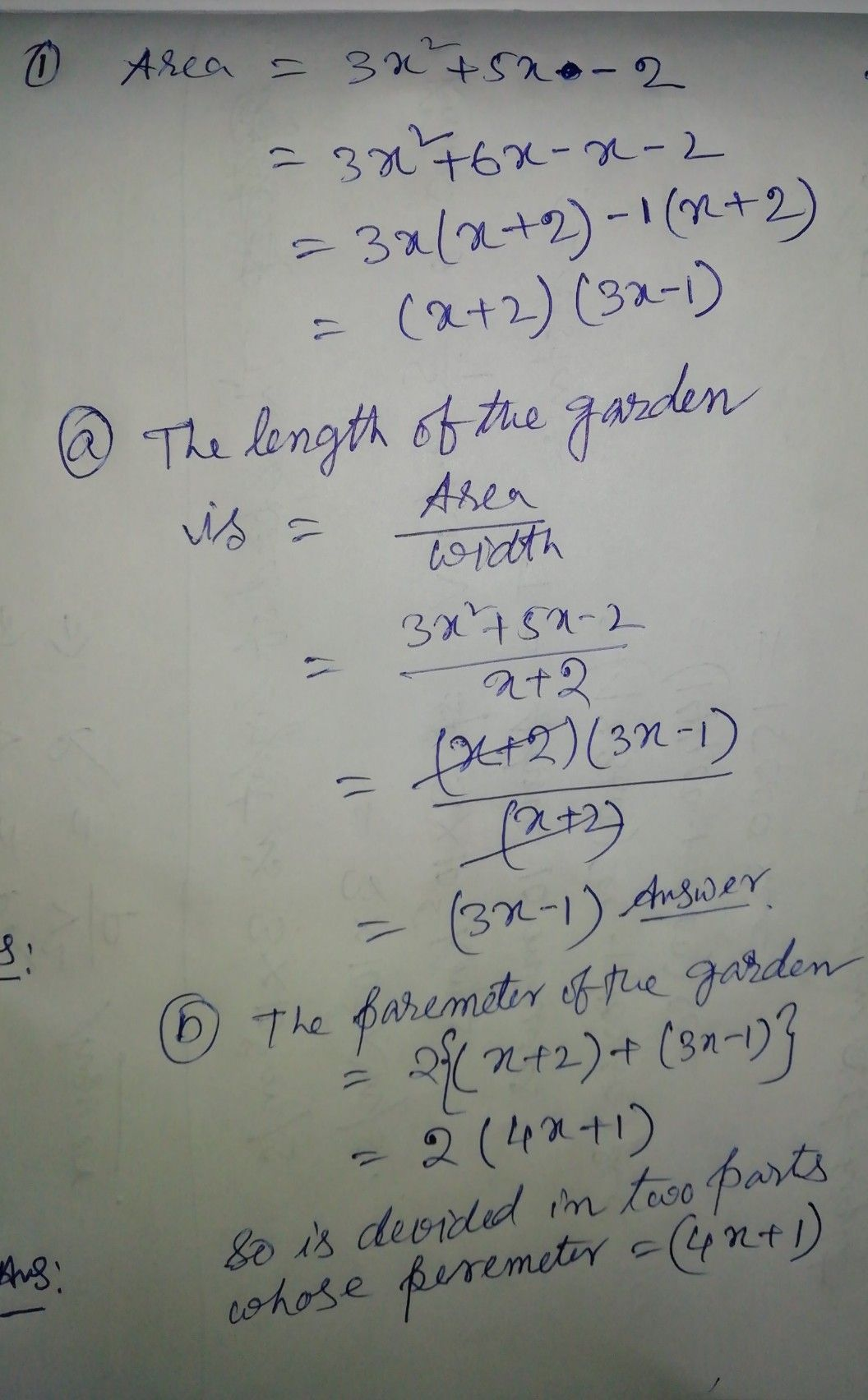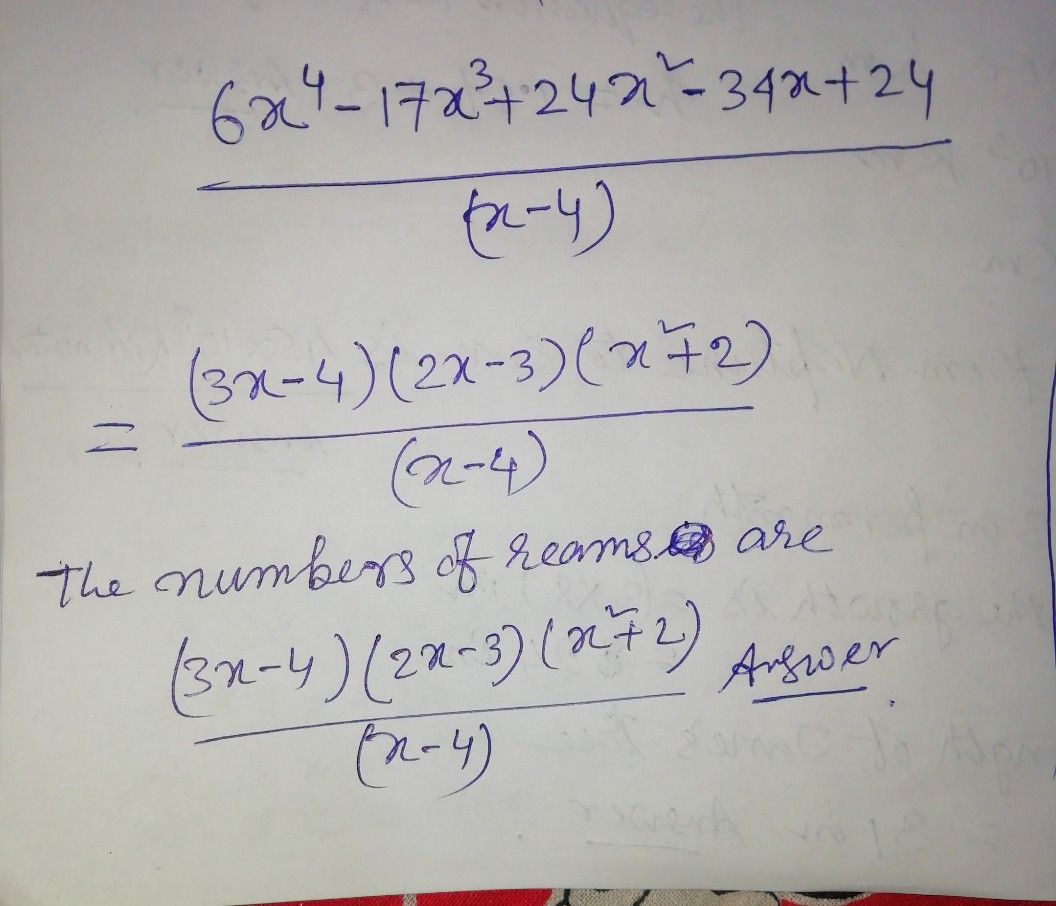Symbol
Problem$13$ Solve the following problems using factoring polynomials. $1,A$ A rectangular garden in a backyard has an area $0|$ $\left(3x^{2+5x-2}\right)$ square $11c$ $tc°$ $1^{°}8$ Its width is $\left(x42\right)$ meters. $a$ Find the length of the garden. $l\right).$ You decided to partition the garden into two or more smaller congruent in gardens. Design a possible model and include mathematical concepts your design. $2.11$ one ream of bond $aP\left(1$ $cos13$ $\left(x-4\right)$ $pcs05$ $101y$ many reams can you buy $lor$ $\left(6x^{4}-17x^{4+24x^{2}-34x+24}\right)$ $pcsos$
10th-13th grade
Other
Search count: 114
SolutionQanda teacher - himadri007any doubt ask me